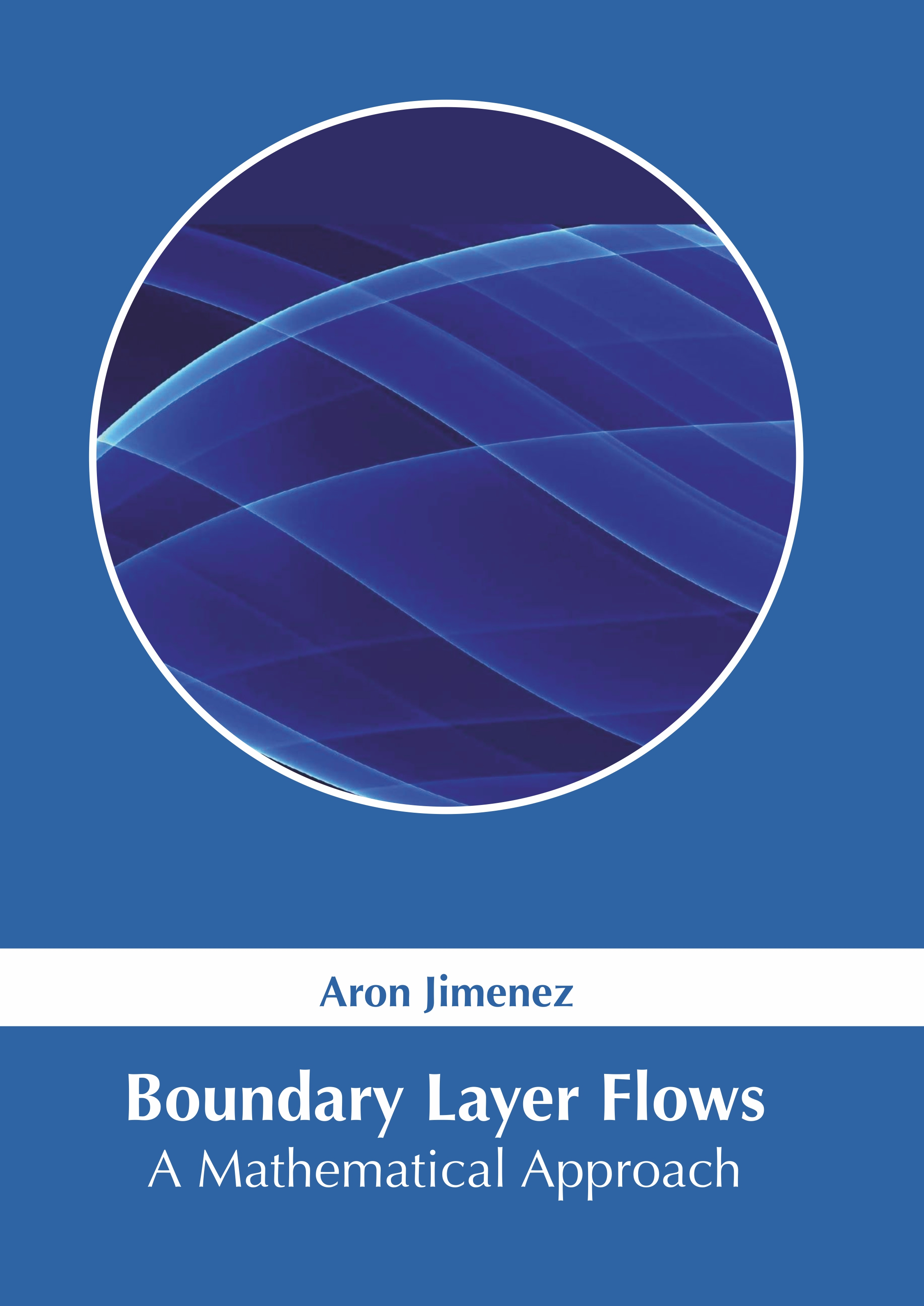# Books /Boundary Layer Flows: A Mathematical Approach

BROWSE BY

### Boundary Layer Flows: A Mathematical Approach9781639890835

Author

Aron Jimenez

English

##### Publication Year

2022

Category :

Mathematics and Statistics - Differential Equations

\$ 143.95

##### Description

The layer of fluid wherein the effects of viscosity are significant and which exists in the immediate vicinity of a bounding surface is known as a boundary layer. Boundary layer equations such as Bernoulli’s equation, Prandtl’s transposition theorem, energy integral, Von Mises transformation, and Crocco’s transformation equations are necessary for the understanding of boundary layer flows. They are also a vital point of fluid dynamics. In mathematical analysis of fluid dynamics, one of the central problems is the asymptotic limit of the fluid flow as viscosity becomes zero. This book unravels the recent studies in the field of boundary layer flows. It will also provide interesting topics for research which interested readers can take up. This book is a vital tool for all researching or studying about boundary layer flows as it gives incredible insights into emerging trends and concepts.